Next: Epipolar geometry Up: Camera model and epipolar Previous: Camera model and epipolar

## Camera model

The camera is modelled by its optical centre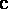and its retinal plane (or image plane). In each camera, a 3-D point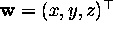in world coordinates (where the world coordinate frame is fixed arbitrarily) is projected into an image pointin camera coordinates, where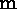is the intersection ofwith the line containing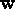and. In projective (or homogeneous) coordinates, the transformation fromtois modelled by the linear transformationwhere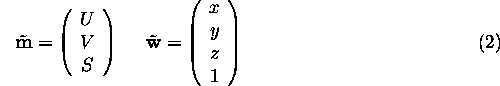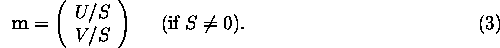The pointsfor which S=0 define the focal plane and are projected to infinity.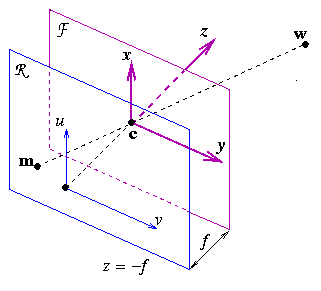Figure 1: Pinhole camera model.

Each pinhole camera is therefore modelled by its perspective projection matrix (PPM), which can be decomposed into the productThe matrix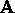gathers the intrinsic parameters of the camera, and has the following form: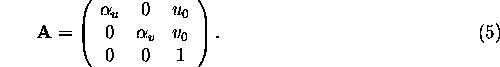whereare the focal lengths in vertical and horizontal pixels, respectively, and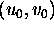are the coordinates of the principal point. The matrix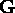is composed by a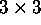rotation matrix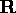and a vector, encoding the camera position and orientation (extrinsic parameters) in the world reference frame, respectively: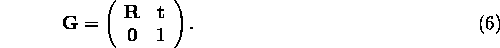Let us write the PPM asThe plane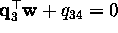(S=0) is the focal plane, and the two planes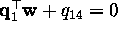and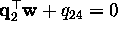intersect the retinal plane in the vertical (U=0) and horizontal (V=0) axis of the retinal coordinates, respectively.

The optical centreis the intersection of the three planes introduced in the previous paragraph; therefore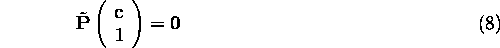and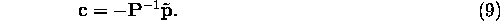The optical ray associated to an image pointis the line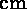, i.e. the set of points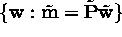. The equation of this ray can be written in parametric form as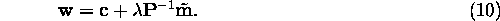withan arbitrary real number.

Andrea Fusiello
Tue Feb 3 17:18:41 MET 1998